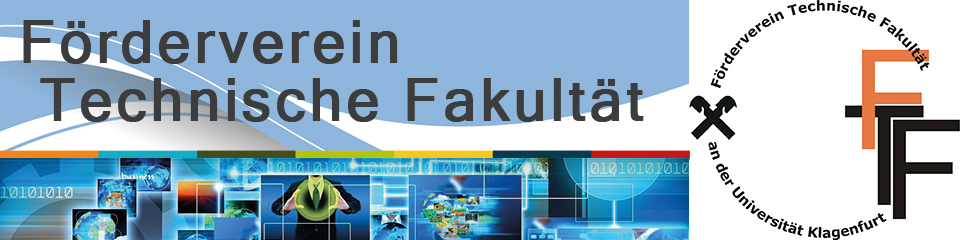## Efficient Algorithms for Hard Problems: Semidefinite Optimization for Binary Quadratic ProgrammingAbstract: Through three prominent combinatorial optimization problems (graph coloring, maximum cut, ordering) we will explain modelling techniques using semidefinite programming (as opposed to linear programming). We will derive relaxations that yield tight bounds and give rise to heuristics to obtain high-quality feasible solutions. We demonstrate how to combine these ingredients within a branch-and-bound framework, thus obtaining an exact solution method.

CV: Angelika Wiegele is a mathematician working in the field of combinatorial optimization and semidefinite optimization. She studied mathematics at the Alpen-Adria-Universit“at and at City University London where she was an Erasmus student